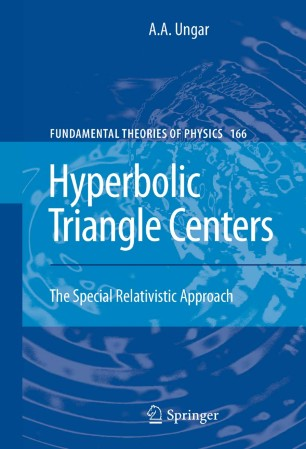# Hyperbolic Triangle Centers

## The Special Relativistic Approach

• A.A. UngarBook

Part of the Fundamental Theories of Physics book series (FTPH, volume 166)

1. Front Matter
Pages I-XVI
2. ### The Special Relativistic Approach To Hyperbolic Geometry

1. Front Matter
Pages 1-1
2. A. A. Ungar
Pages 3-43
3. A. A. Ungar
Pages 45-57
4. A. A. Ungar
Pages 59-81
3. ### Mathematical Tools For Hyperbolic Geometry

1. Front Matter
Pages 83-83
2. A. A. Ungar
Pages 85-115
3. A. A. Ungar
Pages 117-125
4. A. A. Ungar
Pages 127-150
4. ### Hyperbolic Triangle Centers

1. Front Matter
Pages 151-151
2. A. A. Ungar
Pages 153-217
3. A. A. Ungar
Pages 219-267
4. A. A. Ungar
Pages 269-299
5. A. A. Ungar
Pages 301-308
5. Back Matter
Pages 309-319

### Introduction

After A. Ungar had introduced vector algebra and Cartesian coordinates into hyperbolic geometry in his earlier books, along with novel applications in Einstein’s special theory of relativity, the purpose of his new book is to introduce hyperbolic barycentric coordinates, another important concept to embed Euclidean geometry into hyperbolic geometry. It will be demonstrated that, in full analogy to classical mechanics where barycentric coordinates are related to the Newtonian mass, barycentric coordinates are related to the Einsteinian relativistic mass in hyperbolic geometry. Contrary to general belief, Einstein’s relativistic mass hence meshes up extraordinarily well with Minkowski’s four-vector formalism of special relativity. In Euclidean geometry, barycentric coordinates can be used to determine various triangle centers. While there are many known Euclidean triangle centers, only few hyperbolic triangle centers are known, and none of the known hyperbolic triangle centers has been determined analytically with respect to its hyperbolic triangle vertices. In his recent research, the author set the ground for investigating hyperbolic triangle centers via hyperbolic barycentric coordinates, and one of the purposes of this book is to initiate a study of hyperbolic triangle centers in full analogy with the rich study of Euclidean triangle centers. Owing to its novelty, the book is aimed at a large audience: it can be enjoyed equally by upper-level undergraduates, graduate students, researchers and academics in geometry, abstract algebra, theoretical physics and astronomy. For a fruitful reading of this book, familiarity with Euclidean geometry is assumed. Mathematical-physicists and theoretical physicists are likely to enjoy the study of Einstein’s special relativity in terms of its underlying hyperbolic geometry. Geometers may enjoy the hunt for new hyperbolic triangle centers and, finally, astronomers may use hyperbolic barycentric coordinates in the velocity space of cosmology.

### Keywords

Application special relativity Barycentric coordinates Examining hyperbolic triangle center Four-vector Hyperbolic barycentric coordinates Hyperbolic coordinates Hyperbolic geometry Hyperbolic triangle centers Hyperbolic triangle ve Hyperbolic triangle vertex RMS Relativity Special relativity Theoretical physics special theory of relativity

#### Authors and affiliations

• A.A. Ungar
• 1
1. 1.Dept. MathematicsNorth Dakota State UniversityFargoUSA